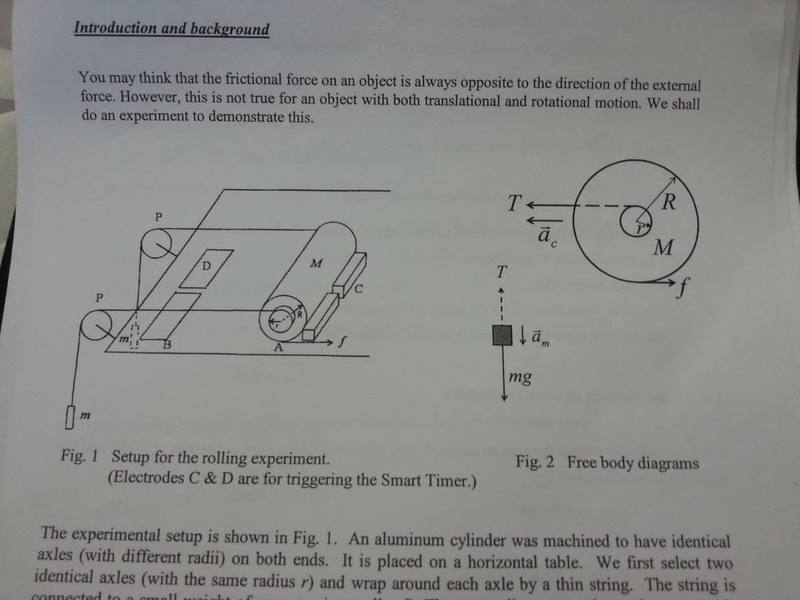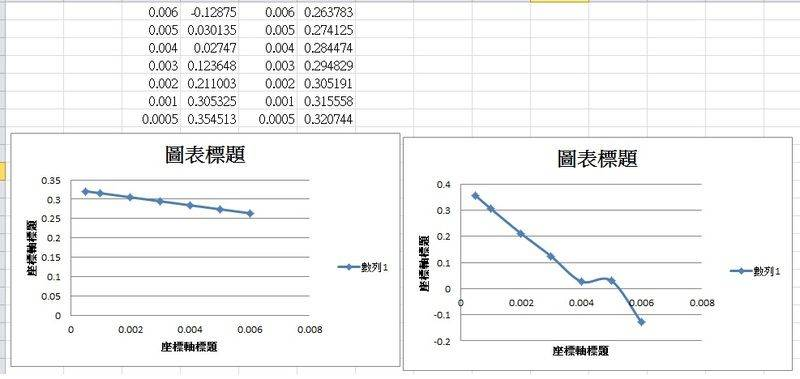# Experiment of frictional force on rolling object

Recently, I have done an experiment of frictional on rolling object.

In the experiment, I have done 7 trials with different axle radii, similar to that of a yoyo on a horizontal table.

the experimental was set as thisAs derived by the lab manual i received, frictional force can be plotted as a function of axle radius.

f=2mgMR(R-2r)/(3MR^2+4m(R+r)^2)

The graph plotted using my experimental data is very strange.......Both graphs are plotted by f as a function of r. The one on the right is plotted according to my experimental data. The one on the left is a plot of the equation above. Why the graphs are so different? Is there anything wrong?

Last edited:

## Answers and Replies

It seems like you have one bad data point in your experimental graph and the plot of the function is incorrect. What were the masses m and M and what is the radius R? The function ought to change signs at r=R/2.

It seems like you have one bad data point in your experimental graph and the plot of the function is incorrect. What were the masses m and M and what is the radius R? The function ought to change signs at r=R/2.

M = 5.743 kg

m = 50.42 g

R = 0.06355 m

i see the problem.......it is a horrible and stupid mistake in changing unit.......thanks for reminding

our professor reminded us that the graph is non-linear and do not have to do fitting

but the graph is fairly linear except the "bad data".

is there any other possible explanation leading to this deviation?

AlephZero
Science Advisor
Homework Helper
but the graph is fairly linear except the "bad data".

is there any other possible explanation leading to this deviation?

A good guess would be that the value +0.030135 should be -0.030135.